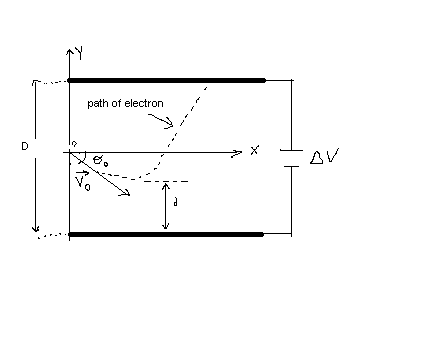Ask question

# Suppose that u,vu,v and w are vectors such that ⟨u,v⟩=6, ⟨v,w⟩=−7, ⟨u,w⟩=13⟨ ∣∣u∣∣=1,∣∣v∣∣=6,∣∣w∣∣=19∣∣u∣∣=1, given expression ⟨u+v,u+w⟩.# Suppose that u,vu,v and w are vectors such that ⟨u,v⟩=6, ⟨v,w⟩=−7, ⟨u,w⟩=13⟨ ∣∣u∣∣=1,∣∣v∣∣=6,∣∣w∣∣=19∣∣u∣∣=1, given expression ⟨u+v,u+w⟩.

Question
Vectorsasked 2021-02-05
Suppose that u,vu,v and w are vectors such that ⟨u,v⟩=6, ⟨v,w⟩=−7, ⟨u,w⟩=13⟨ ∣∣u∣∣=1,∣∣v∣∣=6,∣∣w∣∣=19∣∣u∣∣=1, given expression ⟨u+v,u+w⟩.

## Answers (1)2021-02-06
Given that u,v and w are vectors such that $$\displaystyle{\left({u},{v}\right)}={6},{\left({v},{w}\right)}=-{7},{\left({u},{w}\right)}={13},{\left|{\left|{u}\right|}\right|}={1},{\left|{\left|{v}\right|}\right|}={6},{\left|{\left|{w}\right|}\right|}={19}$$
PSK(u+v,v+w)=(u,v)+(u,w)+(v,v)+(v,w) =6+13+(6^2)-7ZSK =12+36 =48.
Again $$\displaystyle{\left({u}+{v},{u}+{w}\right)}={\left({u},{u}\right)}+{\left({u},{w}\right)}+{\left({v},{u}\right)}+{\left({v},{w}\right)}$$ =1+13+6-7 =13.

### Relevant Questionsasked 2021-03-02

Let U,V be subspaces of Rn. Suppose that $$U\bot V.$$ Prove that $$\{u,v\}$$ is linearly independent for any nonzero vectors $$u\in U,\ v\in V.$$asked 2021-02-24

Let $$u=\begin{bmatrix}2 \\ 5 \\ -1 \end{bmatrix} , v=\begin{bmatrix}4 \\ 1 \\ 3 \end{bmatrix} \text{ and } w=\begin{bmatrix}-4 \\ 17 \\ -13 \end{bmatrix}$$ It can be shown that $$4u-3v-w=0$$. Use this fact (and no row operations) to find a solution to the system $$4u-3v-w=0$$ , where
$$A=\begin{bmatrix}2 & -4 \\5 & 17\\-1&-13 \end{bmatrix} , x=\begin{bmatrix}x_1 \\ x_2 \end{bmatrix} , b=\begin{bmatrix}4 \\ 1 \\ 3 \end{bmatrix}$$asked 2021-02-02
Solve the problem. Let vector u have initial point P1=(0,2) and terminal point P2=(−2,6). Let vector v have initial point Q1=(3,0) and terminal point Q2=(1,4). U and v have the same direction. Find ||u|| and ||v||. Is u=v?asked 2020-10-21
This is the quesetion. Suppose that a does not equal 0.
a. if $$\displaystyle{a}\cdot{b}={a}\cdot{c}$$, does it follow that b=c?
b. if $$\displaystyle{a}\times{b}={a}\times{c}$$, does it follow that b=c ?
c. if $$\displaystyle{a}\cdot{b}={a}\cdot{c}$$ and $$\displaystyle{a}\times{b}={a}\times{c}$$, does it follow that b=c?
Either prove the assertion is true in general or show that it is false for a concret choice of vectors a, b, casked 2020-11-14

If u is an algebraic expression and c is a positive number,
1. The solutions of $$|u| < c$$ are the numbers that satisfy$$-c < u < c$$.
2. The solutions of $$|u| > c$$ are the numbers that satisfy$$u < -c\ or\ u < c$$.
These rules are valid if $$<$$ is replaced be $$\displaystyle\le$$ and $$>$$ is replaced by $$\displaystyle\ge$$asked 2021-04-19
consider the product of 3 functions $$\displaystyle{w}={f}\times{g}\times{h}$$. Find an expression for the derivative of the product in terms of the three given functions and their derivatives. (Remeber that the product of three numbers can be thought of as the product of two of them with the third
$$\displaystyle{w}'=$$?asked 2021-03-02

Let u,$$v_1$$ and $$v_2$$ be vectors in $$R^3$$, and let $$c_1$$ and $$c_2$$ be scalars. If u is orthogonal to both $$v_1$$ and $$v_2$$, prove that u is orthogonal to the vector $$c_1v_1+c_2v_2$$.asked 2020-12-29
The position vector $$\displaystyle{r}{\left({t}\right)}={\left\langle{\ln{{t}}},\frac{{1}}{{t}^{{2}}},{t}^{{4}}\right\rangle}$$ describes the path of an object moving in space.
(a) Find the velocity vector, speed, and acceleration vector of the object.
(b) Evaluate the velocity vector and acceleration vector of the object at the given value of $$\displaystyle{t}=\sqrt{{3}}$$asked 2021-05-12
An electron is fired at a speed of $$\displaystyle{v}_{{0}}={5.6}\times{10}^{{6}}$$ m/s and at an angle of $$\displaystyle\theta_{{0}}=–{45}^{\circ}$$ between two parallel conductingplates that are D=2.0 mm apart, as in Figure. Ifthe potential difference between the plates is $$\displaystyle\triangle{V}={100}\ {V}$$, determine (a) how close d the electron will get to the bottom plate and (b) where the electron will strike the top plate.asked 2020-12-14
Find the vector that has the same direction as (6, 2, -3) but has length 4.
...